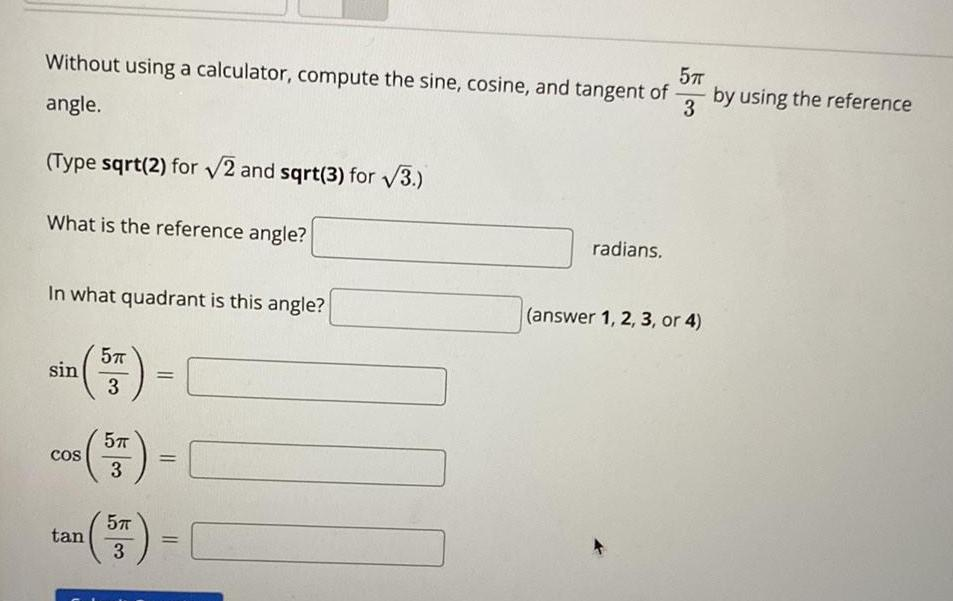Question:

# Without using a calculator, compute the sine, cosine, and

Last updated: 8/2/2022Without using a calculator, compute the sine, cosine, and tangent of 5π/3 by using the reference angle. (Type sqrt(2) for √2 and sqrt(3) for √3.) What is the reference angle? In what quadrant is this angle?